# OpenGL核心技术之Parallax Mapping3D引擎 同时被 2 个专栏收录154 篇文章 15 订阅

CSDN视频网址：http://edu.csdn.net/lecturer/144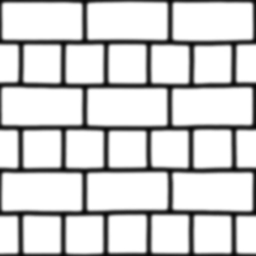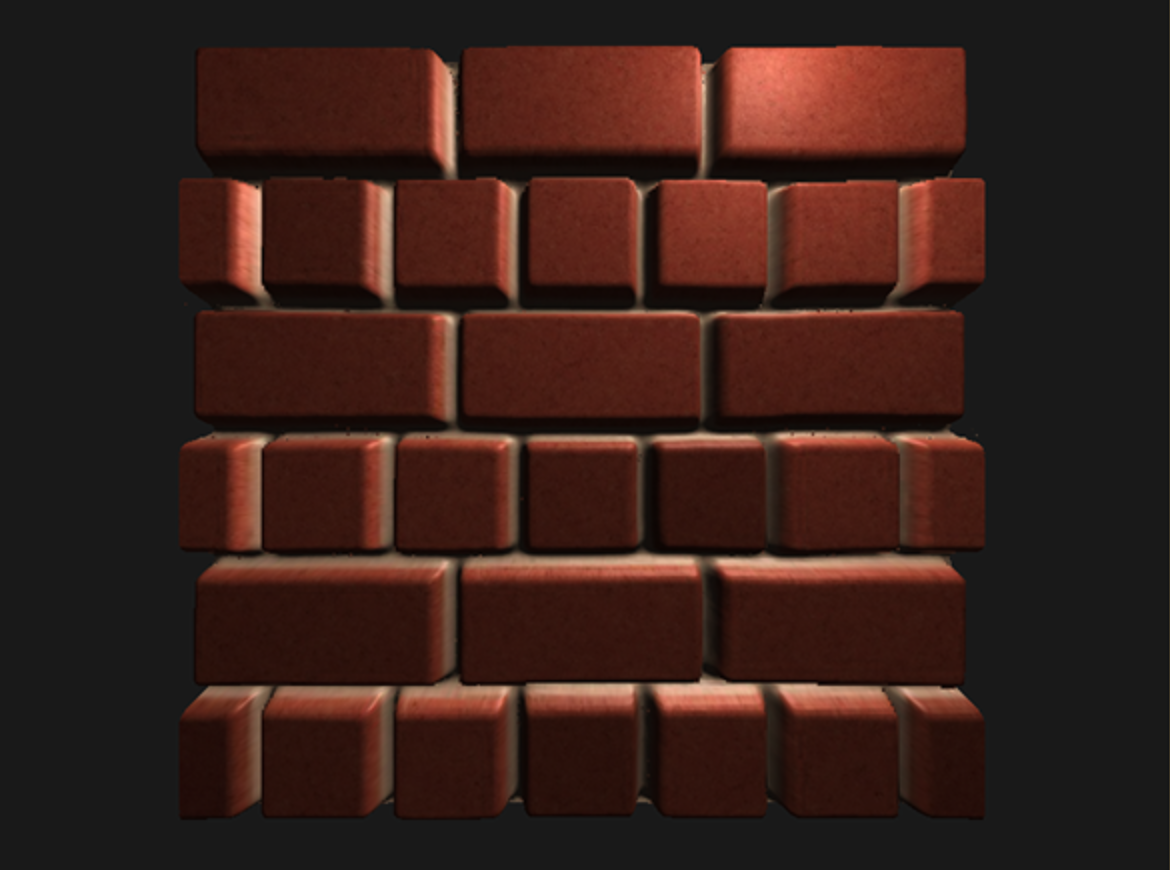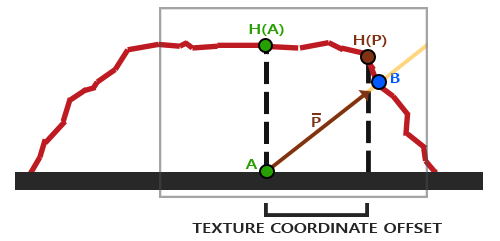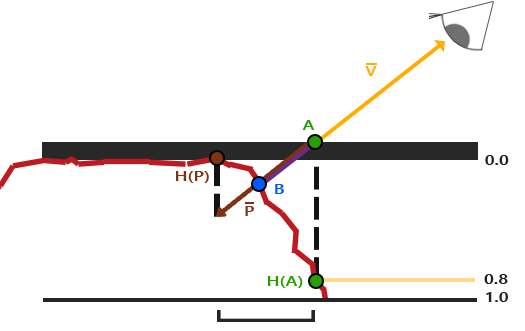#version 330 core
layout (location = 0) in vec3 position;
layout (location = 1) in vec3 normal;
layout (location = 2) in vec2 texCoords;
layout (location = 3) in vec3 tangent;
layout (location = 4) in vec3 bitangent;

out VS_OUT {
vec3 FragPos;
vec2 TexCoords;
vec3 TangentLightPos;
vec3 TangentViewPos;
vec3 TangentFragPos;
} vs_out;

uniform mat4 projection;
uniform mat4 view;
uniform mat4 model;

uniform vec3 lightPos;
uniform vec3 viewPos;

void main()
{
gl_Position      = projection * view * model * vec4(position, 1.0f);
vs_out.FragPos   = vec3(model * vec4(position, 1.0));
vs_out.TexCoords = texCoords;

vec3 T   = normalize(mat3(model) * tangent);
vec3 B   = normalize(mat3(model) * bitangent);
vec3 N   = normalize(mat3(model) * normal);
mat3 TBN = transpose(mat3(T, B, N));

vs_out.TangentLightPos = TBN * lightPos;
vs_out.TangentViewPos  = TBN * viewPos;
vs_out.TangentFragPos  = TBN * vs_out.FragPos;
}

#version 330 core
out vec4 FragColor;

in VS_OUT {
vec3 FragPos;
vec2 TexCoords;
vec3 TangentLightPos;
vec3 TangentViewPos;
vec3 TangentFragPos;
} fs_in;

uniform sampler2D diffuseMap;
uniform sampler2D normalMap;
uniform sampler2D depthMap;

uniform float height_scale;

vec2 ParallaxMapping(vec2 texCoords, vec3 viewDir);

void main()
{
// Offset texture coordinates with Parallax Mapping
vec3 viewDir   = normalize(fs_in.TangentViewPos - fs_in.TangentFragPos);
vec2 texCoords = ParallaxMapping(fs_in.TexCoords,  viewDir);

// then sample textures with new texture coords
vec3 diffuse = texture(diffuseMap, texCoords);
vec3 normal  = texture(normalMap, texCoords);
normal = normalize(normal * 2.0 - 1.0);
// proceed with lighting code
[...]
}

vec2 ParallaxMapping(vec2 texCoords, vec3 viewDir)
{
float height =  texture(depthMap, texCoords).r;
vec3 p = viewDir.xy / viewDir.z * (height * height_scale);
return texCoords - p;
}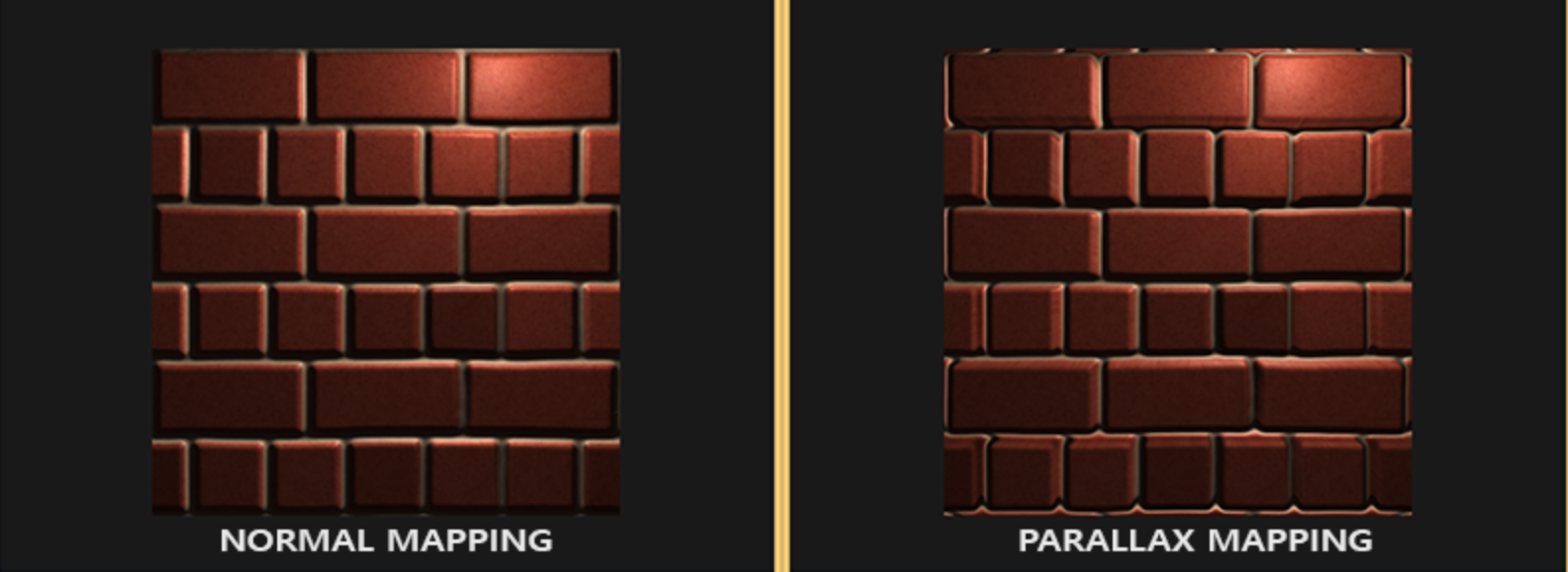texCoords = ParallaxMapping(fs_in.TexCoords,  viewDir);
if(texCoords.x > 1.0 || texCoords.y > 1.0 || texCoords.x < 0.0 || texCoords.y < 0.0)
discard;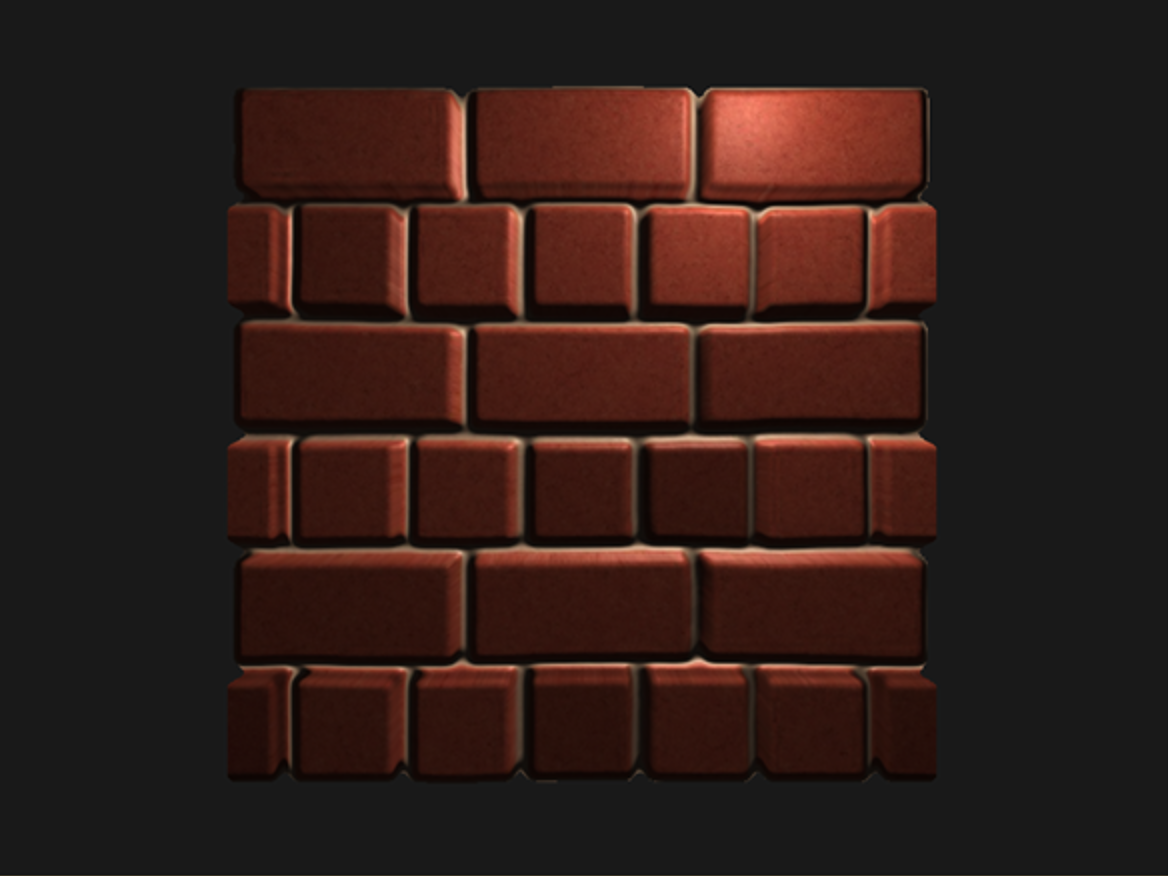#version 330 core
layout (location = 0) in vec3 position;
layout (location = 1) in vec3 normal;
layout (location = 2) in vec2 texCoords;
layout (location = 3) in vec3 tangent;
layout (location = 4) in vec3 bitangent;

out VS_OUT {
vec3 FragPos;
vec2 TexCoords;
vec3 TangentLightPos;
vec3 TangentViewPos;
vec3 TangentFragPos;
} vs_out;

uniform mat4 projection;
uniform mat4 view;
uniform mat4 model;

uniform vec3 lightPos;
uniform vec3 viewPos;

void main()
{
gl_Position = projection * view * model * vec4(position, 1.0f);
vs_out.FragPos = vec3(model * vec4(position, 1.0));
vs_out.TexCoords = texCoords;

vec3 T = normalize(mat3(model) * tangent);
vec3 B = normalize(mat3(model) * bitangent);
vec3 N = normalize(mat3(model) * normal);
mat3 TBN = transpose(mat3(T, B, N));

vs_out.TangentLightPos = TBN * lightPos;
vs_out.TangentViewPos  = TBN * viewPos;
vs_out.TangentFragPos  = TBN * vs_out.FragPos;
}

#version 330 core
out vec4 FragColor;

in VS_OUT {
vec3 FragPos;
vec2 TexCoords;
vec3 TangentLightPos;
vec3 TangentViewPos;
vec3 TangentFragPos;
} fs_in;

uniform sampler2D diffuseMap;
uniform sampler2D normalMap;
uniform sampler2D depthMap;

uniform bool parallax;
uniform float height_scale;

vec2 ParallaxMapping(vec2 texCoords, vec3 viewDir)
{
float height =  texture(depthMap, texCoords).r;
return texCoords - viewDir.xy / viewDir.z * (height * height_scale);
}

void main()
{
// Offset texture coordinates with Parallax Mapping
vec3 viewDir = normalize(fs_in.TangentViewPos - fs_in.TangentFragPos);
vec2 texCoords = fs_in.TexCoords;
if(parallax)
texCoords = ParallaxMapping(fs_in.TexCoords,  viewDir);

// Obtain normal from normal map
vec3 normal = texture(normalMap, texCoords).rgb;
normal = normalize(normal * 2.0 - 1.0);

// Get diffuse color
vec3 color = texture(diffuseMap, texCoords).rgb;
// Ambient
vec3 ambient = 0.1 * color;
// Diffuse
vec3 lightDir = normalize(fs_in.TangentLightPos - fs_in.TangentFragPos);
float diff = max(dot(lightDir, normal), 0.0);
vec3 diffuse = diff * color;
// Specular
vec3 reflectDir = reflect(-lightDir, normal);
vec3 halfwayDir = normalize(lightDir + viewDir);
float spec = pow(max(dot(normal, halfwayDir), 0.0), 32.0);

vec3 specular = vec3(0.2) * spec;
FragColor = vec4(ambient + diffuse + specular, 1.0f);
}

04-03
08-178102-061131
12-233079
05-308220
03-1990
03-2664
08-2426
12-161180
04-07361
08-081714
02-0397
04-205146
09-181796
08-0871
04-0755
10-28229
04-28415点击重新获取扫码支付余额充值